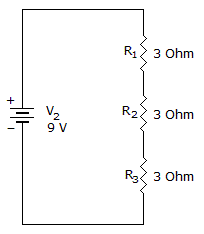# Electronics - Series Circuits - Discussion

### Discussion :: Series Circuits - General Questions (Q.No.1)

1.

What is the current flow through R1, R2, and R3?[A]. 1A, 1A, 1A [B]. 1A, 2A, 3A [C]. 3A, 3A, 3A [D]. 3A, 2A, 1A

Explanation:

V = IR

V = I(R1 + R2 + R3)

I = V/(R1 + R2 + R3)

= 9/(3 + 3 + 3)

= 9/9

= 1 Amp.

 Surya said: (Jul 17, 2011) Same current flows in series ckt.

 Krishna said: (Dec 13, 2011) Current will be same across 3 resistor as they connected in series.

 Manteshkumar said: (Mar 30, 2012) Yes its correct because resistors are in series thats why same current will flow.

 Luca said: (Jan 5, 2013) Yes, in fact, series resistors vary only the voltage, but not the current, whilst, if the resistors were placed in parallel among each other, the current will change and the voltage will remain unchanged.

 Veerababu said: (Sep 19, 2013) I = V/R. If V = 9V; R = R1+R2+R3. = 3+3+3. = 9. V = 9; R = 9. I = V/R. I = 9/9. I = 1 Amp.

 Dakpo Saviour Dela Doyen said: (May 14, 2014) The effective resistance in the circuit is 9 ohms and the emf is 9volts. By Ohm's law, I=V/R =9/9=1 A. Since components are in series with the voltage source the same current of 1A flow through them.

 Lizzy Mametja said: (Nov 12, 2014) The answer is A because Rs. 9 and the voltage is 9 so we use I = V/R and then you will get your answer.

 Saifuddin said: (Nov 18, 2016) The total resistance of the circuit is 9 ohm, so by ohm law I= Vs/Rt. 9v/9ohm = 1amp.

 Raju said: (Dec 7, 2017) I = V/R. V = 9V; R = 3+3+3. = 9. V = 9; R = 9. I = V/R. I = 9/9. I = 1 Amp.